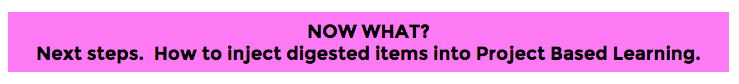# 129: Creativity & Beauty of MathematicsTraditional School Math & Math Misconceptions
• Math is hated/feared because it is taught and thought of in ways that are different from other subjects.
• Primary role of students in traditional math classrooms is to perform and get questions right.
• Performing takes precedence over learning.
• Testing culture promotes idea that math is about finding short answers to narrow questions under pressure.
• Math is a subject of procedures, calculations and rules.
• Math is a dead subject that is only present in textbook calculations.
• Math is a series of answers to questions that nobody asks in the real world.
• Math in classrooms focuses primary on Stage 3 of math stages (see below)
• Student shouldn’t have to show work if their answer is right.
• Math is done by individuals.
• People who are good at math perform calculations QUICKLY.  Math is a speed race.

Real Nature of Mathematics
• Besides getting question right, doing math involves:
• appreciating beauty of math
• thinking deeply
• exploring math connections
• applying math to different situations
• exploring patterns
• using math to create and analyze new technology and strategies
• formulating questions
• Math exists throughout nature, art, and the world.
• Nature contains many examples of mathematics
• spiders are experts in spirals
• dolphins use a form of algebra to interpret echolocation signals
• Instead of study of procedures, calculations and rules, math is
• study of patterns (aesthetic, beauty of subject)
• subject of visual images, creativity and connections
• subject that is full of uncertainty – answers can be explorations, interpretations, conjectures
• set of ideas, connections and relationships that we can use to make sense of the world
• 4 Stages of Math Work
1. Posing a question
2. Going from real world to a mathematical model
3. Performing a calculation
4. Going from model back to real world to see if original question was answered
• Real math is often done collaboratively
• Speed of calculations has nothing to do with math fluency.

How to Align Math Schooling with Real Mathematics
• Give students opportunities to consider situation and formulate math problems to investigate these situations
• Give students opportunities to use all 4 math stages
• Require students to show work because displaying logical mathematical lines of reasoning is the main part of doing math
• Facilitate math discussions about mathematical reasoning (what it is, how to critique and justify it)
• Pose open-end problems and allow students to develop methods and pathways to solutions

Workforce Implications
• Employers need people who can ask good questions, set up models, analyze results and interpret mathematical answers.
• Employers no longer need people to calculate; now they need people to think and reasonMath misconceptions have crept into the design of math curricula.  These math misconceptions have made the subject appear uninteresting and unappealing to some.  Knowing the true nature of mathematics can help teachers design learning experiences for students that are engaging, challenging and relevant to the real world.Preparation Steps
• Research more methods for designing learning experiences that are more true to the math discipline.  See Mathematics articles.
• Research strategies for 4 Phases of Math (see above).
• Create a culture that values mistakes.  See this article for ideas.
• Design scaffolding that includes elements such as:
• balance of 4 phases of math
• facilitated math discussions about mathematical reasoning
• pattern recognition
• students posing questions and possible solutions
• student creating models for the real world
Early Implementation Steps
• Implement scaffolding that provides students with many opportunities to appreciate and practice real mathematical thinking
• Have students reflect on how their math attitudes are changing as a result of math activities that deliberately mimic the math discipline